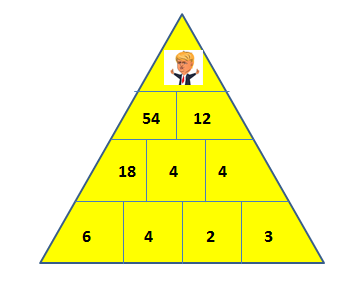top of page

# Brain scratching math riddle of the day

Solve the following math exercise in under 2 minutes if you are a genius:

Find the number that should be in place of Donald Trump in the following pyramid.GOOD LUCK!

.

.

.

.

.

.

.

.

.

.

```Answer: 594

The numbers in the concerned row are the product of the first two boxes in the preceding row minus the number in the first box.
E.g.
SECOND ROW NUMBERS
6*4-6=18
4*2-4=4
2*3-2=4

THIRD ROW NUMBERS
18*4-18=54
4*4-4=12

54*12-54=594

```